normal distribution

(redirected from Normal distribution curve)
Also found in: Dictionary, Thesaurus, Medical, Financial, Acronyms.

normal distribution

[′nȯr·məl ‚di·strə′byü·shən]
(statistics)
A commonly occurring probability distribution that has the form where e is the mean and σ is the variance. Also known as Gauss' error curve; Gaussian distribution.

normal distribution

a continuous distribution of a random VARIABLE with its mean, median and mode equal (see MEASURES OF CENTRAL TENDENCY). Thus the normal curve is symmetrical, and bell-shaped as in Fig. 21 below. See also PROBABILITY, PARAMETRIC STATISTICS assume the parent population to have a normal distribution. In reality, a normal distribution is only approximated, and this is regarded as acceptable to fulfil this requirement of a parametric test.

Normal Distribution

one of the most important probability distributions. The term “normal distribution” refers both to probability distributions of random variables and to joint probability distributions of several random variables, that is, to distributions of random vectors.

The probability distribution of a random variable X is said to be normal if it has a probability density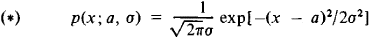The family of normal distributions (*) thus depends on the two parameters a and σ. Here, the expected value of X is equal to a and the variance of X is equal to σ2. The graph of the normal density Y= p(x; a,σ) is symmetric with respect to the ordinate, which passes through the point x = a, and has at this point a unique maximum equal to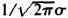. The normal density curve becomes increasingly narrow as σ decreases (see Figure 1). Changing a with σ held constant does not alter the form of the curve but causes it to be displaced along the abscissa. The area under the normal curve is always equal to unity. When a = 0 and σ = 1, the corresponding distribution function is equal to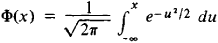In the general case, the normal distribution function F(x; a,σ) can be calculated by the formula F (x; a, σ) = Φ (t), where t = (x — a)/σ. Extensive tables have been compiled for the function Φ(t) and for several derivatives of the function. For a normal distribution, the probability that the inequality ǀX — a ǀ > kσ will be satisfied, which is equal to 1 — Φ(k) + Φ(–k), decreases extremely rapidly as k increases (see Table 1). The possibility of deviations from a that exceed 3σ is therefore disregarded in many practical problems involving a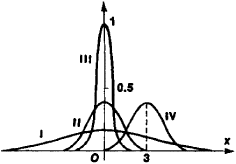Figure 1. Normal-distribution density curves for various values of the parameters a and σ : (I) a = 0, σ = 2.5; (II) a = 0, σ = 1; (III) a = 0, σ = 0.4; (IV) a = 3, σ = 1

normal distribution—the three-σ rule. (As can be seen from Table 1, the corresponding probability is less than 0.003.) The probable deviation for the normal distribution is 0.67449σ

Table 1
kProbability
1 .....................................0.31731
2 .....................................0.04550
3 .....................................0.00269
4 .....................................0.00006

Normal distributions are encountered in a large number of applications. Attempts to explain this have long been made. Theoretical justification of the exceptional role of the normal distribution is given by the limit theorems in probability. The relevant result can be qualitatively explained in the following manner. The normal distribution serves as a good approximation whenever the random variable under consideration represents the sum of a large number of independent random variables, with the largest variable being small in comparison with the sum.

The normal distribution may also appear as an exact solution for some problems—within the framework of the mathematical model that is used for the problem. This is the case in the theory of random processes (in one of the principal models of Brownian motion). Classical examples of the normal distribution as an exact distribution were given by K. Gauss (the distribution of errors of observations) and J. Maxwell (the distribution of molecular velocities).

The joint distribution of several random variables X1, X2, …, Xs is said to be a multivariate normal distribution if the corresponding probability density has the form

p(x1, …, xn) = C exp[–Q(x1 - a1, …, xs - as)]

where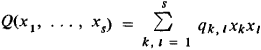in which qk, l = ql, k is a positive definite quadratic form. The constant C is determined from the condition that the integral of p over all space be equal to unity. The parameters a1, …, as are equal to the expected values of X1, …, Xs, respectively, and the coefficient qk, l can be expressed in terms of the variances σ21,: . ., σ2s of these variables and the correlation coefficient pk, l for Xk and Xi. The total number of parameters defining a normal distribution is equal to

(s + 1)(s + 2)/2 - 1

and increases rapidly with s; the number of parameters equals 2, 20, and 65, respectively, when s =1,5, and 10. The multivariate normal distribution is the basic model for multidimensional statistical analysis. It is also used in the theory of random processes, where the normal distribution in infinite-dimensional spaces is also considered.

For information on problems associated with the estimation of normal-distribution parameters on the basis of observational results, seeSMALL SAMPLES and UNBIASED ESTIMATE. For verification of the assumption of normality, seeNONPARAMETRIC METHODS (in mathematical statistics).

IU. V. PROKHOROV

normal distribution

(statistics)
(Or "Gaussian distribution") The frequency distribution of many natural phenomena such as the height or intelligence of people of a certain age and sex. The formula looks something like:

P(x) = e^(((x-m)/s)^2)

where P(x) is the probability of a measurement x, m is the mean value of x and s is the standard deviation.

Also known as a "bell curve" because of its shape.
References in periodicals archive ?
They include: the number of samples with prevailing snowflake type; the percentage corresponding to the number of snow elements in snowflakes, N(SCSF), divided by the total number of snow elements (individuals and in the snowflakes), Ntot; mean and maximum number of snow crystals in a snowflake (SC in SF); the mean sizes of snowflakes corresponding to the accumulated mean size occurrences (assuming usually the applicability of the combination of two normal distribution curves); size of the snowflake and the accumulated percentage corresponding to the slope change in the normal distribution curve.
The general shape of the distribution of real estate returns can be seen in Figure 1, which shows the normal distribution curve superimposed on histograms of actual returns.
"The normal distribution curve is symmetrical, where most of the observations cluster around the central peak and the probabilities for values further away from the mean taper off equally in both directions.
Figures 7(a), 7(b) and 7(c) present the normal distribution curves and percent of the population in a given range for both the tooth diameter and inter-tooth spacing values using their means and standard deviations.
"Rugged Logic allows you to get down to a considerable amount of detail and statistical analysis such as normal distribution curves.
In contrast to the normal distribution curves shown in Figure 1b, the average and median values in Figure 2b are not equal because a significant part of the area under the curve is contributed by large values of x relatively far out on the right-hand tail.

Site: Follow: Share:
Open / Close